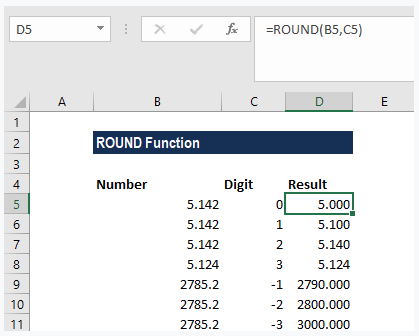# What Is The ROUND Function?

The ROUND Function is categorized under Excel Math and Trigonometry functions and it rounds up a number to a specified number of digits.

Unlike the ROUNDUP and ROUNDDOWN functions, the ROUND function can round either up or down.

Discover the best online rounding calculators.

## ROUND Function Formula

=ROUND(number,num_digits)

The function uses the following arguments:

#1: Number (required argument) – This is a real number that we wish to round.

#2: Num_digits (required argument) – This is the number of digits to which we want to round the number.

Now if the num_digits argument is:

1. A positive value that is greater than zero, it specifies the number of digits to the right of the decimal point.
2. Equal to zero, it specifies rounding to the nearest integer.
3. A negative value that is less than zero, it specifies the number of digits to the left of the decimal point.

Rounding numbers – hardware implementations and implications.

## How To Use The ROUND Function In Excel?

To understand the uses of the ROUND function, let’s consider a few examples:

Example #1:

Suppose we are given the following data:

The results we get in Excel are shown below:

Example #2:

Let’s see how to round prices using the function to the nearest 0.99 value. Suppose we are given the following data:

The formula used is shown below:

The ROUND function would first round 63.39 to 63 and then subtract 0.01 to give 62.99. So, the function with a zero would round the number given to the nearest whole dollar. Once it is rounded, the formula would simply subtract 0.01 from the number to get a .99 value.

We get the result below:

Understanding the decimal place value.

## The ROUNDDOWN Function

The ROUNDDOWN Function rounds down towards zero.

## The ROUNDDOWN Function Formula

=ROUNDDOWN(number,num_digits)

The ROUNDDOWN function uses the following arguments:

#1: Number (required argument) – This is a real number that we wish to round down.

#2: Num_digits (required argument) – This is the number of digits to which we want to round the number.

Now, if the num_digits argument is:

1. A positive value that is num_digits > number, it specifies the number of digits is rounded down to the specified number of decimal places.
2. Equal to zero, it specifies rounding to the nearest integer.
3. A negative value that is num_digits < number, it specifies the number of digits is rounded down to the specified number of decimal places.

## The ROUNDUP Function

The ROUNDUP Function rounds up a number away from zero.

## ROUNDUP Function Formula

=ROUNDUP(number,num_digits)

The ROUNDUP function uses the following arguments:

#1: Number (required argument) – This is a real number that we wish to round up.

#2: Num_digits (required argument) – This is the number of digits to which we want to round the number.

Now, if the num_digits argument is:

1. A positive value, it specifies the number of digits to the right of the decimal point.
2. Equal to zero, it specifies rounding to the nearest integer.
3. A negative value, it specifies the number of digits to the left of the decimal point.